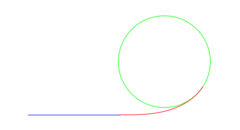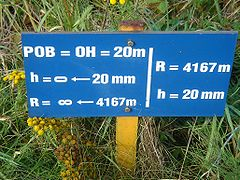# Track transition curve

﻿
Track transition curveThe red Euler spiral is an example of an easement curve between a blue straight line and a circular arc, shown in green.This sign aside a railroad (between Ghent and Bruges) indicates the start of the transition curve. A parabolic curve (POB) is used.

A track transition curve, or spiral easement, is a mathematically calculated curve on a section of highway, or railroad track, where a straight section changes into a curve. It is designed to prevent sudden changes in centripetal force. In plan (i.e., the horizontal curve) the start of the transition is at infinite radius and at the end of the transition it has the same radius as the curve itself, thus forming a very broad spiral. At the same time, in the vertical plane, the outside of the curve is gradually raised until the correct degree of bank is reached.

If such easement were not applied, the lateral acceleration of a rail vehicle would change abruptly at one point – the tangent point where the straight track meets the curve – with undesirable results. With a road vehicle the driver naturally applies the steering alteration in a gradual manner and the curve is designed to permit this, using the same principle.

## History

On early railroads, because of the low speeds and wide-radius curves employed, the surveyors were able to ignore any form of easement, but during the 19th century, as speeds increased, the need for a track curve with gradually increasing curvature became apparent. Rankine's 1862 "Civil Engineering" cites several such curves, including an 1828 or 1829 proposal based on the "curve of sines" by William Gravatt, and the curve of adjustment by William Froude around 1842 approximating the elastic curve. The actual equation given in Rankine is that of a cubic curve, which is a polynomial curve of degree 3. This was also known as cubic parabola at that time.

In the UK, only from 1845 when legislation and land costs began to constrain the laying out of rail routes and tighter curves were necessary, did the principles start to be applied in practice.

The "true spiral", where the curvature is exactly linear in arclength, requires more sophisticated mathematics (in particular, the ability to integrate its intrinsic equation) to compute than the proposals cited by Rankine. Several late-19th century civil engineers seem to have derived the equation for this curve independently (all unaware of the original characterization of this curve by Leonhard Euler in 1744). Charles Crandall gives credit to one Ellis Holbrook, in the Railroad Gazette, Dec. 3, 1880, for the first accurate description of the curve. Another early publication was The Railway Transition Spiral by Arthur N. Talbot, originally published in 1890. Some early 20th century authors call the curve "Glover's spiral", attributing it to James Glover's 1900 publication.

The equivalence of the railroad transition spiral and the clothoid seems to have been first published in 1922 by Arthur Lovat Higgins. Since then, "clothoid" is the most common name given the curve, even though the correct name (following standards of academic attribution) is "the Euler spiral".

## Geometry

While railroad track geometry is intrinsically three-dimensional, for practical purposes the vertical and horizontal components of track geometry are usually treated separately.

The overall design pattern for the vertical geometry is typically a sequence of constant grade segments connected by vertical transition curves in which the local grade varies linearly with distance and in which the elevation therefore varies quadratically with distance. Here grade refers to the tangent of the angle of rise of the track. The design pattern for horizontal geometry is typically a sequence of straight line (i.e., a tangent) and curve (i.e. a circular arc) segments connected by transition curves.

In a tangent segment the track bed roll angle is typically zero. In the case of railroad track the track roll angle (cant or camber) is typically expressed as the difference in elevation of the two rails, a quantity referred to as the superelevation. A track segment with constant non-zero curvature will typically be superelevated in order to have the component of gravity in the plane of the track provide a majority of the centripetal acceleration inherent in the motion of a vehicle along the curved path so that only a small part of that acceleration needs to be accomplished by lateral force applied to vehicles and passengers or lading. The change of superelevation from zero in a tangent segment to the value selected for the body of a following curve occurs over the length of a transition curve that connects the tangent and the curve proper. Over the length of the transition the curvature of the track will also vary from zero at the end abutting the tangent segment to the value of curvature of the curve body, which is numerically equal to one over the radius of the curve body.

The simplest and most commonly used form of transition curve is that in which the superelevation and horizontal curvature both vary linearly with distance along the track. Cartesian coordinates of points along this spiral are given by the Fresnel integrals. The resulting shape matches a portion of an Euler spiral, which is also commonly referred to as a clothoid, and sometimes Cornu spiral.

A transition curve can connect a track segment of constant non-zero curvature to another segment with constant curvature that is zero or non-zero of either sign. Successive curves in the same direction are sometimes called progressive curves and successive curves in opposite directions are called reverse curves.

The Euler spiral has two advantages. One is that it is easy for surveyors because the coordinates can be looked up in Fresnel integral tables. The other is that it provides the shortest transition subject to a given limit on the rate of change of the track superelevation (i.e. the twist of the track). However, as has been recognized for a long time, it has undesirable dynamic characteristics due to the large (conceptually infinite) roll acceleration and rate of change of centripetal acceleration at each end. Because of the capabilities of personal computers it is now practical to employ spirals that have dynamics better than those of the Euler spiral.

Wikimedia Foundation. 2010.

### Look at other dictionaries:

• Track (rail transport) — Permanent way redirects here. For other uses, see Permanent way (disambiguation). Twin rail tracks in a wooded area …   Wikipedia

• Minimum railway curve radius — 90 foot (27.43 m) radii on the elevated 4 ft 8 1⁄2 in (1,435 mm) Chicago L . There is no room for longer radii at this street intersection The minimum railway curve radius, the shortest design radius, has an… …   Wikipedia

• Euler spiral — A double end Euler spiral. An Euler spiral is a curve whose curvature changes linearly with its curve length (the curvature of a circular curve is equal to the reciprocal of the radius). Euler spirals are also commonly referred to as spiros,… …   Wikipedia

• Degree of curvature — This article is about the measure of curvature. For other uses, see degree (angle). Degree of curve or degree of curvature is a measure of curvature of a circular arc used in civil engineering for its easy use in layout surveying. A n degree… …   Wikipedia

• Glossary of rail terminology — Rail transport Operations Track Maintenance High speed Gauge Stations …   Wikipedia

• Railroad switch — For other uses, see Switch (disambiguation). A right hand railroad switch with point indicator pointing to right …   Wikipedia

• List of mathematics articles (T) — NOTOC T T duality T group T group (mathematics) T integration T norm T norm fuzzy logics T schema T square (fractal) T symmetry T table T theory T.C. Mits T1 space Table of bases Table of Clebsch Gordan coefficients Table of divisors Table of Lie …   Wikipedia

• Rail profile — Rail from 1896 Cross sections of flat bottomed which can rest directly …   Wikipedia

• Cant (road/rail) — The cant of a road (sometimes referred to as camber or cross slope) or railway (also referred to as superelevation) is the difference in elevation between the two edges. A cant which is not equal to zero results in a banked turn, allowing… …   Wikipedia